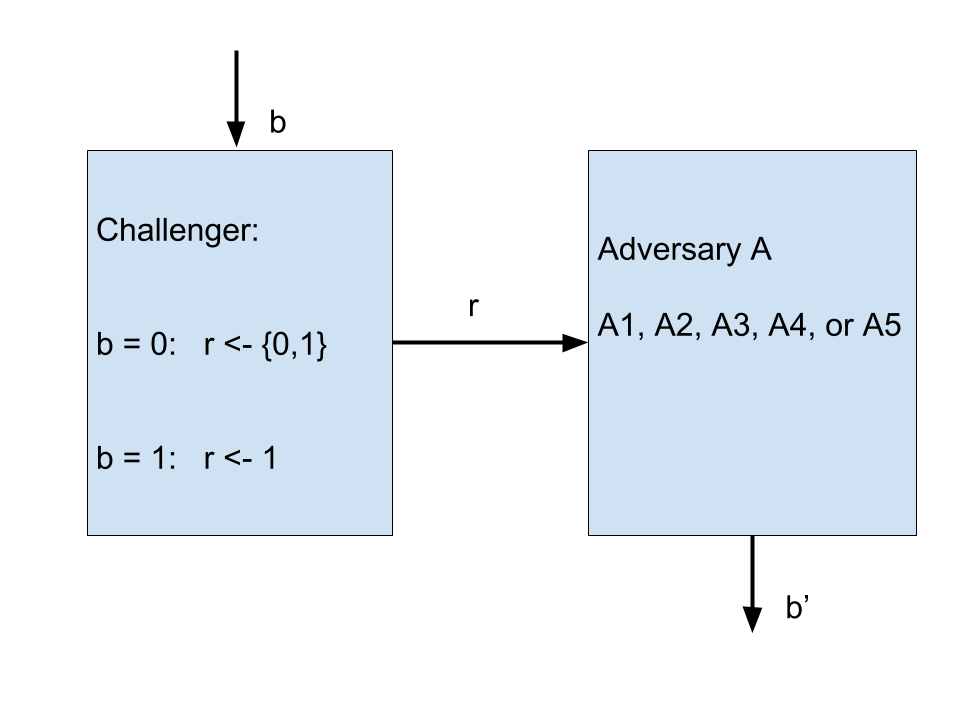## Lab 04 - PRGs, PRFs and PRPs

In this lab we shall do some exercises related to PRFs, PRPs and DES. Please check the course, available here: http://cs.curs.pub.ro/2014/pluginfile.php/13095/mod_resource/content/1/sasc_curs4.pdf

### Exercise 1 (4p)

Advantage. The purpose of this problem is to clarify the concept of advantage. Consider the following two experiments $\mathsf{EXP}(0)$ and $\mathsf{EXP}(1)$:

• In $\mathsf{EXP}(0)$ the challenger flips a fair coin (probability $1/2$ for HEADS and $1/2$ for TAILS) and sends the result to the adversary $\mathsf{A}$.
• In $\mathsf{EXP}(1)$ the challenger always sends TAILS to the adversary.

Let r = 0 for HEADS and r = 1 for TAILS. Then we have the experiment as shown below:The adversary’s goal is to distinguish these two experiments: at the end of each experiment the adversary outputs a bit $0$ or $1$ for its guess for which experiment it is in. For $b = 0,1$ let $W_{b}$ be the event that in experiment $b$ the adversary output $1$. The adversary tries to maximize its distinguishing advantage, namely the quantity $\mathsf{Adv} = \left| \mathsf{Pr}\left[W_{0}\right] − \mathsf{Pr}\left[W_{1}\right] \right| \in \left[0, 1\right]$ .

The advantage $\mathsf{Adv}$ captures the adversary’s ability to distinguish the two experiments. If the advantage is $0$ then the adversary behaves exactly the same in both experiments and therefore does not distinguish between them. If the advantage is $1$ then the adversary can tell perfectly what experiment it is in. If the advantage is negligible for all efficient adversaries (as defined in class) then we say that the two experiments are indistinguishable.

• A1: Always output $1$.
• A2: Ignore the result reported by the challenger, and randomly output $0$ or $1$ with even probability.
• A3: Output $1$ if HEADS was received from the challenger, else output $0$.
• A4: Output $0$ if HEADS was received from the challenger, else output $1$.
• A5: If HEADS was received, output $1$. If TAILS was received, randomly output $0$ or $1$ with even probability.

### Exercise 2 (4p)

Let $F : K × X \to Y$ be a secure PRF with $K = X = Y = \{0, 1\}^{n}$.

• a) Show that $F_1(k,x) = F(k,x) \| 0$ is not a secure PRF. (for strings $y$ and $z$ we use $y \| z$ to denote the concatenation of $y$ and $z$)
• b) Prove that $F_2(k, x) = F \left(k, x \oplus 1^{n}\right)$ is a secure PRF. Here $x \oplus 1^{n}$ is the bit-wise complement of $x$. To prove security argue the contra-positive: a distinguisher $A$ that breaks $F_2$ implies a distinguisher $B$ that breaks $F$ and whose running time is about the same as $A$’s.
• c) Let $K_3 = \{0, 1\}^{n+1}$. Construct a new PRF $F_3 : K_3 \times X \to Y$ with the following property: the PRF $F_3$ is secure, however if the adversary learns the last bit of the key then the PRF is no longer secure. This shows that leaking even a single bit of the secret key can completely destroy the PRF security property.

Hint: Let $k_3 = k \| b$ where $k \in \{0,1\}^{n}$ and $b \in \{0,1\}$. Set $F_3(k_3,x)$ so that $F_3$ is a secure PRF, but becomes easily distinguishable from a random function if the last bit of the secret key $k_3$ is known to the adversary. Prove that your $F_3$ is a secure PRF by arguing the contra-positive, as in part (b).

### Exercise 3 (2p)

Let $F : K × X \to Y$ be a secure PRF with $K = X = Y = \{0, 1\}^{n}$.

Give example of a secure PRG $G : K \to Z$ with $Z = \{0, 1\}^{nt}$.

### Exercise 4 (2p)

In class we showed the indistinguishability game, which we used to determine the advantage of an adversary against an encryption scheme that wants to provide semantic security (i.e. against an eavesdropper that observes a single ciphertext). In the game, the adversary sends two messages, $m_0$ and $m_1$ and gets to see the encryption $c_b = E(k, m_b)$ of one of them (depending on the random bit $b$). The adversary's task is to determine correctly which message was encrypted by returning a bit $b'$.

We defined security of encryption scheme E against an eavesdropper in two ways:

• $Pr[A(c_b)=b] \le \frac{1}{2} + negl(n)$
• $|Pr[A(c_1)=1] - Pr[A(c_0)=1]| \le negl(n)$

where $A(c_i) = j$ means that when the adversary receives the encryption of message $i$ he returns the bit $b'=j$.

Show that the two are equivalent.